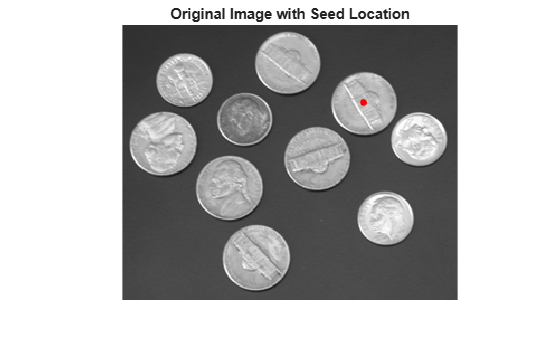Calculate weights for image pixels based on image gradient

## Syntax

``W = gradientweight(I)``
``W = gradientweight(I,sigma)``
``W = gradientweight(___,Name,Value)``

## Description

````W = gradientweight(I)` calculates the pixel weight for each pixel in image `I` based on the gradient magnitude at that pixel, and returns the weight array `W`. The weight of a pixel is inversely related to the gradient values at the pixel location. Pixels with small gradient magnitude (smooth regions) have a large weight and pixels with large gradient magnitude (such as on the edges) have a small weight.```
````W = gradientweight(I,sigma)` uses `sigma` as the standard deviation for the derivative of Gaussian that is used for computing the image gradient.```

example

````W = gradientweight(___,Name,Value)` returns the weight array `W` using name-value pairs to control aspects of weight computation.```

## Examples

collapse all

This example segments an image using the Fast Marching Method based on the weights derived from the image gradient.

```I = imread('coins.png'); imshow(I) title('Original Image')```Compute weights based on image gradient.

```sigma = 1.5; W = gradientweight(I, sigma, 'RolloffFactor', 3, 'WeightCutoff', 0.25);```

Select a seed location.

```R = 70; C = 216; hold on; plot(C, R, 'r.', 'LineWidth', 1.5, 'MarkerSize',15); title('Original Image with Seed Location')```Segment the image using the weight array.

```thresh = 0.1; [BW, D] = imsegfmm(W, C, R, thresh); figure, imshow(BW) title('Segmented Image') hold on; plot(C, R, 'r.', 'LineWidth', 1.5, 'MarkerSize',15);```Geodesic distance matrix `D` can be thresholded using different thresholds to get different segmentation results.

```figure, imshow(D) title('Geodesic Distances') hold on; plot(C, R, 'r.', 'LineWidth', 1.5, 'MarkerSize',15);```## Input Arguments

collapse all

Grayscale image, specified as a numeric matrix.

Data Types: `single` | `double` | `int8` | `uint8` | `int16` | `uint16` | `int32` | `uint32`

Standard deviation for derivative of Gaussian, specified as a positive number.

Data Types: `double`

### Name-Value Arguments

Specify optional pairs of arguments as `Name1=Value1,...,NameN=ValueN`, where `Name` is the argument name and `Value` is the corresponding value. Name-value arguments must appear after other arguments, but the order of the pairs does not matter.

Before R2021a, use commas to separate each name and value, and enclose `Name` in quotes.

Example: `W = gradientweight(I,1.5,'RolloffFactor',3,'WeightCutoff',0.25);`

Output weight roll-off factor, specified as the comma-separated pair consisting of `'RolloffFactor'` and a positive scalar of class `double`. Controls how fast weight values fall as a function of gradient magnitude. When viewed as a 2-D plot, pixel intensity values might vary gradually at the edges of regions, creating a gentle slope. In your segmented image, you might want the edge to be more well-defined. Using the roll-off factor, you control the slope of the weight value curve at points where intensity values start to change. If you specify a high value, the output weight values fall off sharply around the edges of smooth regions. If you specify a low value, the output weight has a more gradual fall-off around the edges. The suggested range for this parameter is ```[0.5 4]```.

Data Types: `double`

Threshold for weight values, specified as the comma-separated pair consisting of `'WeightCutoff'` and a positive number in the range `[1e-3 1]`. If you use this parameter to set a threshold on weight values, it suppresses any weight values less than the value you specify, setting these pixels to a small constant value (1e-3). This parameter can be useful in improving the accuracy of the output when you use the output weight array `W` as input to Fast Marching Method segmentation function, `imsegfmm`.

Data Types: `double`

## Output Arguments

collapse all

Weight array, returned as a numeric array of the same size as the input image, `I`. The weight array is of class `double`, unless `I` is `single`, in which case it is of class `single`.

## Tips

• `gradientweight` uses double-precision floating point operations for internal computations for all classes of `I`, except when `I` is of class `single`, in which case `gradientweight` uses single-precision floating point operations internally.

## Version History

Introduced in R2014b